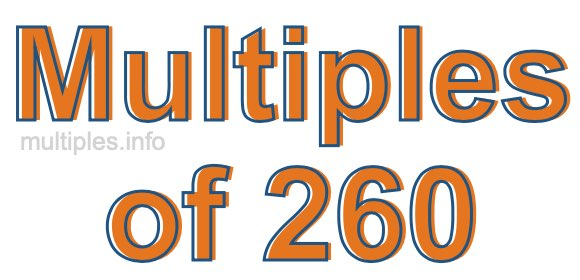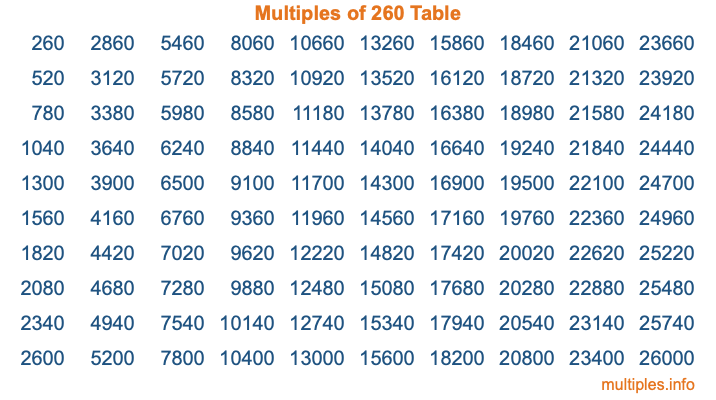Multiples of 260Welcome to the Multiples of 260 page. Here we will first teach you everything you will ever need to know about the multiples of 260, and then give you a study guide summary of everything we taught you to make sure you remember it all. Use this page to look up facts and learn information about the multiples of 260. This page will make you a multiples of two hundred sixty expert!

Definition of Multiples of 260
Multiples of 260 are all the numbers that when divided by 260 equal an integer. Each of the multiples of 260 are called a multiple. A multiple of 260 is created by multiplying 260 by an integer.

Therefore, to create a list of multiples of 260, you start with 1 multiplied by 260, then 2 multiplied by 260, then 3 multiplied by 260, and so on for as long as you want. Thus, the list of the first five multiples of 260 is 260, 520, 780, 1040, and 1300. To see a larger list of multiples of 260, see the printable image of Multiples of 260 further down on this page. We also have a category where you can choose any nth multiple of 260.

Multiples of 260 Checker
The Multiples of 260 Checker below checks to see if any number of your choice is a multiple of 260. In other words, it checks to see if there is any number (integer) that when multiplied by 260 will equal your number. To do that, we divide your number by 260. If the the quotient is an integer, then your number is a multiple of 260.

Is  a multiple of 260?

Least Common Multiple of 260 and ...
A Least Common Multiple (LCM) is the lowest multiple that two or more numbers have in common. This is also called the smallest common multiple or lowest common multiple and is useful to know when you are adding our subtracting fractions. Enter one or more numbers below (260 is already entered) to find the LCM.

Check out our LCM Calculator if you need more details about the Least Common Multiple or if you need the LCM for different numbers for adding and subtraction fractions.

nth Multiple of 260
As we stated above, 260 is the first multiple of 260, 520 is the second multiple of 260, 780 is the third multiple of 260, and so on. Enter a number below to find the nth multiple of 260.

th multiple of 260

Multiples of 260 vs Factors of 260
260 is a multiple of 260 and a factor of 260, but that is where the similarities end. All postive multiples of 260 are 260 or greater than 260. All positive factors of 260 are 260 or less than 260.

Below is the beginning list of multiples of 260 and the factors of 260 so you can compare:

Multiples of 260: 260, 520, 780, 1040, 1300, etc.

Factors of 260: 1, 2, 4, 5, 10, 13, 20, 26, 52, 65, 130, 260

As you can see, the multiples of 260 are all the numbers that you can divide by 260 to get a whole number. The factors of 260, on the other hand, are all the whole numbers that you can multiply by another whole number to get 260.

It's also interesting to note that if a number (x) is a factor of 260, then 260 will also be a multiple of that number (x).

Multiples of 260 vs Divisors of 260
The divisors of 260 are all the integers that 260 can be divided by evenly. Below is a list of the divisors of 260.

Divisors of 260: 1, 2, 4, 5, 10, 13, 20, 26, 52, 65, 130, 260

The interesting thing to note here is that if you take any multiple of 260 and divide it by a divisor of 260, you will see that the quotient is an integer.

Multiples of 260 Table
Below is an image of the first 100 multiples of 260 in a table. The table is in chronological order, column by column. The first column has the first ten multiples of 260, the second column has the next ten multiples of 260, and so on.The Multiples of 260 Table is also referred to as the 260 Times Table or Times Table of 260. You are welcome to print out our table for your studies.

Negative Multiples of 260
Although not often discussed or needed in math, it is worth mentioning that you can make a list of negative multiples of 260 by multiplying 260 by -1, then by -2, then by -3, and so on, to get the following list of negative multiples of 260:

-260, -520, -780, -1040, -1300, etc.

Multiples of 260 Summary
Below is a summary of important Multiples of 260 facts that we have discussed on this page. To retain the knowledge on this page, we recommend that you read through the summary and explain to yourself or a study partner why they hold true.

There are an infinite number of multiples of 260.

A multiple of 260 divided by 260 will equal a whole number.

260 divided by a factor of 260 equals a divisor of 260.

The nth multiple of 260 is n times 260.

The largest factor of 260 is equal to the first positive multiple of 260.

260 is a multiple of every factor of 260.

260 is a multiple of 260.

A multiple of 260 divided by a divisor of 260 equals an integer.

260 divided by a divisor of 260 equals a factor of 260.

Any integer times 260 will equal a multiple of 260.

Multiples of a Number
Here you can get the multiples of another number, all with the same attention to detail as we did for multiples of 260 on this page.

Multiples of
Multiples of 261
Did you find our page about multiples of two hundred sixty educational? Do you want more knowledge? Check out the multiples of the next number on our list!